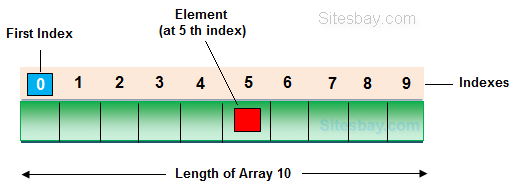# Array In Java

## Array in java

Array is a collection of similar type of data. It is fixed in size means that you can't increase the size of array at run time. It is a collection of homogeneous data elements. It stores the value on the basis of the index value.One variable can store multiple value: The main advantage of the array is we can represent multiple value under the same name.

Code Optimization: No, need to declare a lot of variable of same type data, We can retrieve and sort data easily.

Random access: We can retrieve any data from array with the help of the index value.

The main limitation of the array is Size Limit when once we declare array there is no chance to increase and decrease the size of an array according to our requirement, Hence memory point of view array concept is not recommended to use. To overcome this limitation in Java introduce the collection concept.

## Types of Array in Java

There are two types of array in Java.

• Single Dimensional Array
• Multidimensional Array

## Array Declaration

Single dimension array declaration.

## Syntax

```1.   int[]  a;
2.   int  a[];
3.   int  []a;
```

Note: At the time of array declaration we cannot specify the size of the array. For Example int a; this is wrong.

2D Array declaration.

## Syntax Array in Java

```1.   int[][]  a;
2.   int  a[][];
3.   int  [][]a;
4.   int[]  a[];
5.   int[]  []a;
6.   int   []a[];
```

## Array creation

Every array in a Java is an object, Hence we can create array by using new keyword.

## Syntax

```int[] arr = new int;    // The size of array is 10.
or
int[] arr = {10,20,30,40,50};
```

## Accessing array elements

Access the elements of array by using index value of an elements.

## Syntax Array in Java

```arrayname[n-1];
```

## Access Array Elements

```int[] arr={10,20,30,40};
System.out.println("Element at 4th place"+arr);
```

## Example of Array

```public class ArrayEx
{
public static void main(String []args)
{
int arr[] = {10,20,30};
for (int i=0; i < arr.length; i++)
{
System.out.println(arr[i]);
}
}
}
```

## Output

```10
20
39
```

Note:

1) At the time of array creation we must be specify the size of array otherwise get an compile time error. For Example
int[] a=new int[]; Invalid.
int[] a=new int; Valid

2) If we specify the array size as negative int value, then we will get run-time error, NegativeArraySizeException.

3) To specify the array size the allowed data types are byte, short, int, char If we use other data type then we will get an Compile time error.

4) The maximum allowed size of array in Java is 2147483647 (It is the maximum value of int data type)

## Difference Between Length and Length() in Java

length: It is a final variable and only applicable for array. It represent size of array.

## Example

```int[] a=new int;
System.out.println(a.length);  // 10
System.out.println(a.length());  // Compile time error
```

length(): It is the final method applicable only for String objects. It represents the number of characters present in the String.

## Example

```String s="Java";
System.out.println(s.length());  // 4
System.out.println(s.length);  // Compile time error
```